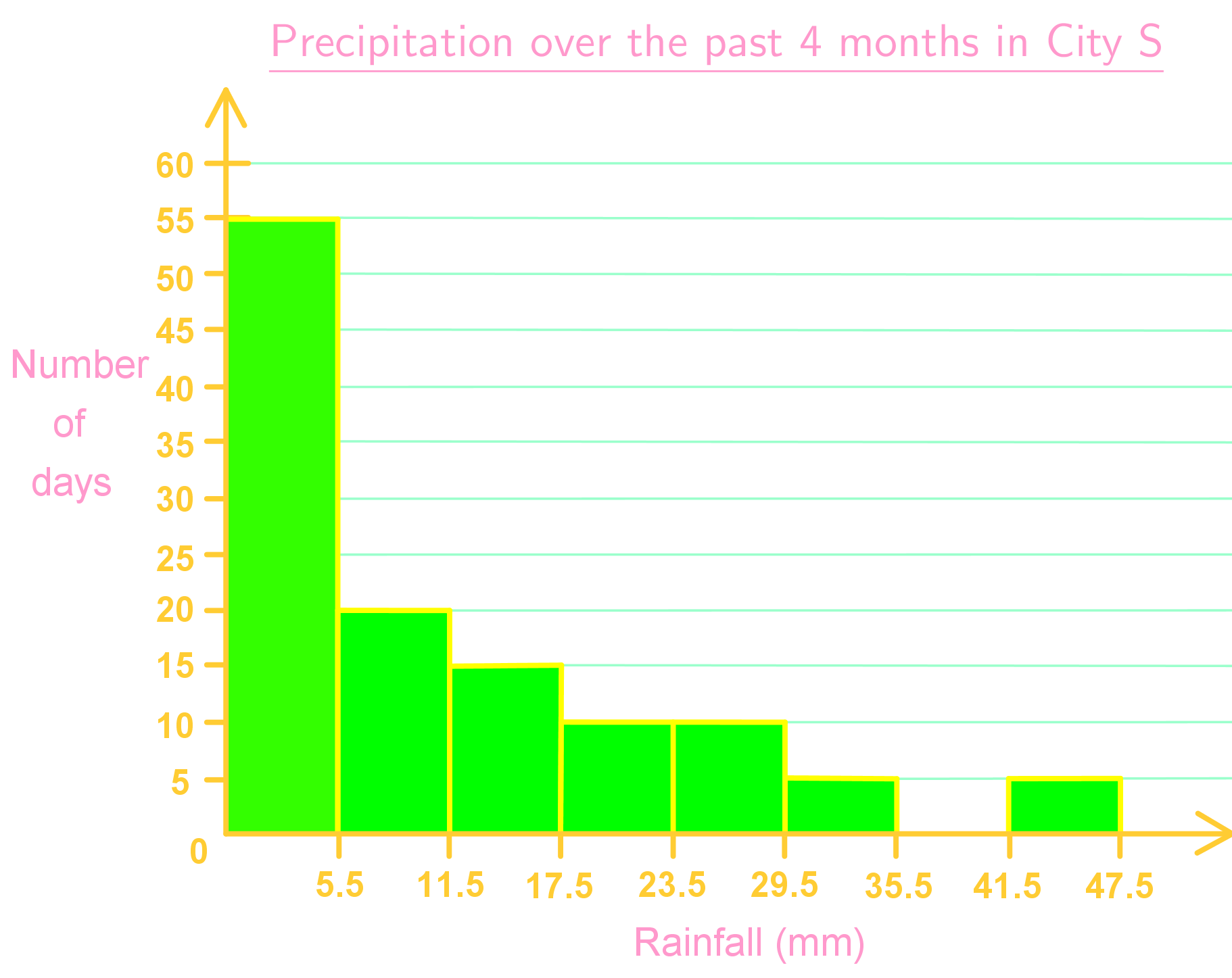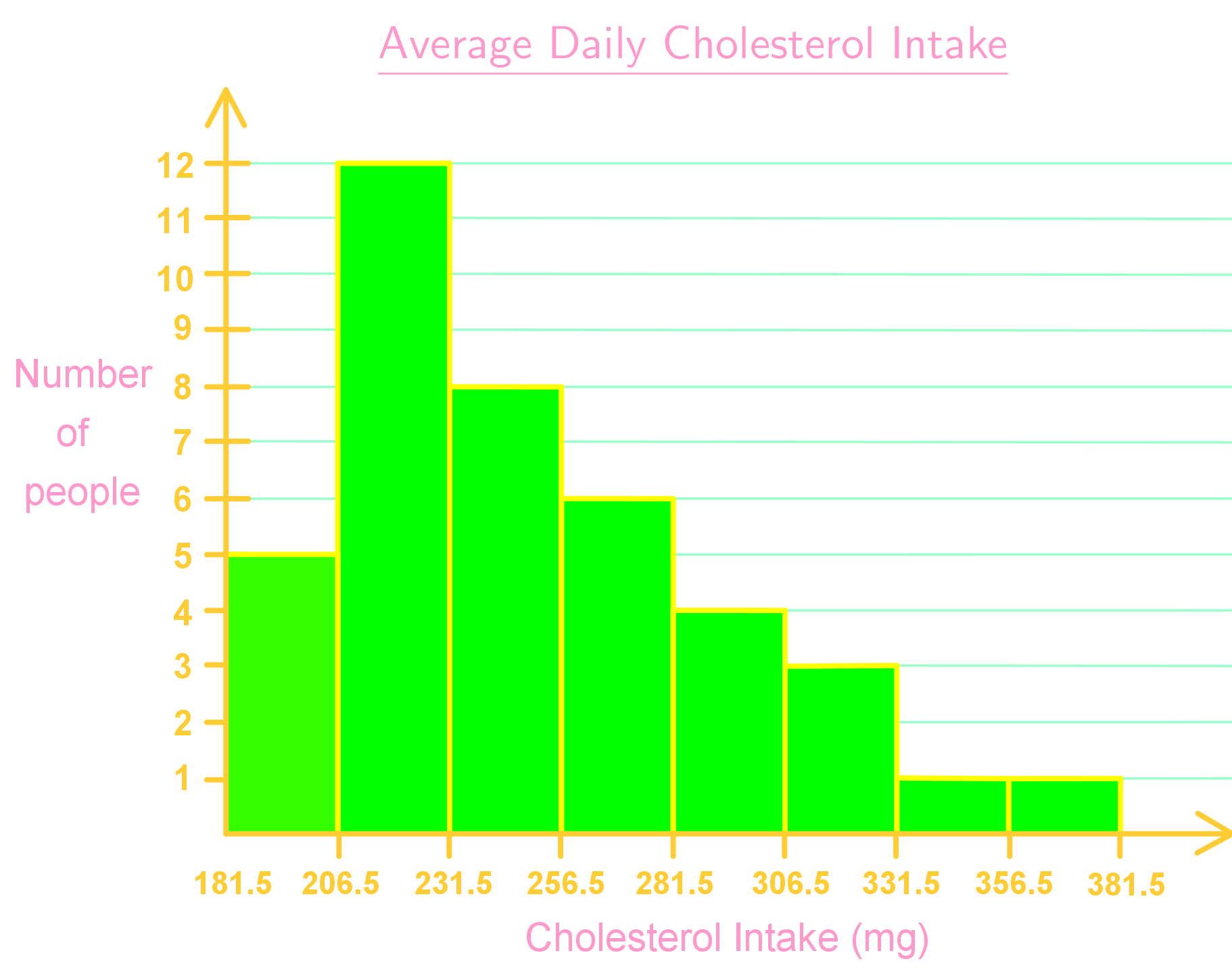# Frequency distribution and histograms

## What is frequency distribution?

Frequency is a measure of how many times a certain event occurs. In math and data, we may have lists of numbers after carrying out research. Amongst the list, certain numbers may occur more than once. Therefore, the frequency of that number happens more than once. When you list out the different frequencies in a table, you'll get a frequency distribution table!

To put the frequency distribution definition into more mathematical terms, frequency distribution is a way to orderly sort data based on the magnitude of the observations. It's a way to summarize and present data effectively.

We're going to be dealing with sorting numbers into frequency distribution tables in this lesson. Before we do that, we'll teach you some terms that you'll need to reference when it comes to frequency distribution tables. They are the following.

Classes: Observations are usually classified into groups called classes or a class interval.

Class Limits: This refers to the smallest to largest possible values in a class. For example, if the smallest number in a class was 5 and the largest was a 25, then the class limit are 5 and 25. In this case, 5 is called the lower class limit and the 25 is the upper class limit.

Midpoint of each class: This is the mid value of each class and can be found with the formula:

(lower limit + upper limit)/2

Magnitude of a class interval: This shows the difference between the lower and upper limit of a class. For our previous example with a lower class limit of 5 and an upper class limit of 25, the magnitude would've been 20.

Class frequency: This is the number of times an observation of an event happens in a class interval.

Keeping the above terms in mind, you can now properly explain different aspects of a frequency distribution table.

## How to find frequency distribution

What info do we need to know in order to find frequency distribution and make a frequency table? Let's pretend that there are several restaurants: Sobway, MacDoonalds, and Burger Kong. You then go ahead and ask you friends which between the three they like better.

Let's say 7 of your friends like Sobway. 9 of your friends respond that they like MacDoonalds. The remaining 4 said that they liked Burger Kong.

 Restaurant Number of friends who prefer restaurant Sobway ||||| || MacDoonalds ||||| |||| Burger Kong ||||

As you can see, this table above is a good way to represent how many of your friends like which restaurants. You can see clearly how many people like each of the different restaurants all in one place. This is actually a frequency distribution table! It can tell you how many things fit into a certain class. Here, our classes refer to our restaurants.

With this info, you can then do more things with the data. For example, displaying the data in a histogram, pie chart, or line chart are some of the many methods you can use to show the information in a visual manner. This will be explored more in future lessons.

### Frequency distribution and histograms

#### Lessons

• Introduction
What is a frequency distribution? Why is it important?

• 1.
Constructing Frequency Distribution Table and Histograms
Listed below are the heights of a class of $7^{th}$ graders:
 119 116 120 134 119 117 115 122 125 124 128 122 135 126 135 120 112 119 115 124 120 117 123 140 125 130 113 128 125 115 131 122 120 138 116

a)
Construct a frequency distribution table based on the data set above with the following information:
1) class (set class width as 4 and lower limits of the classes as multiples of 4)
2) frequency
3) relative frequency
4) cumulative frequency

b)
From the frequency distribution table in the previous part, construct a histogram. Use class midpoints on $x$-axis and frequency on $y$-axis.

• 2.
Interpreting Histograms
Answer the questions based on the histogram below.a)
How many days had more than 35.5 mm of rainfall?

b)
How many more days had 11.5 to 17.5 mm of rainfall than between 35.5 to 47.5 mm of rainfall?

c)
What is the relative frequency of having 17.5 to 23.5 mm of rainfall?

• 3.
The histogram below shows the average daily cholesterol intake of 40 people.a)
What will change in the histogram if class midpoints are used on $x$-axis?

b)
What will change in the histogram if relative frequency is used on $y$-axis?

c)
What will change in the histogram if cumulative frequency is used on $y$-axis?

d)
What kind of interpretations can be made from the cumulative frequency histogram?